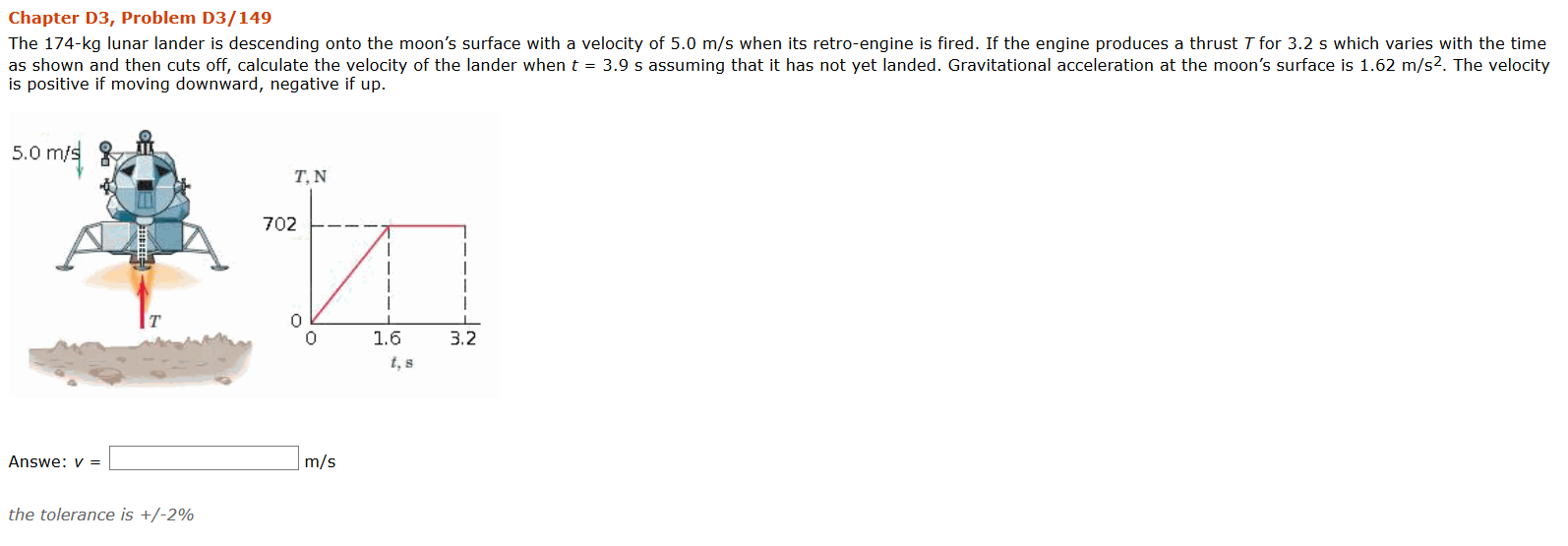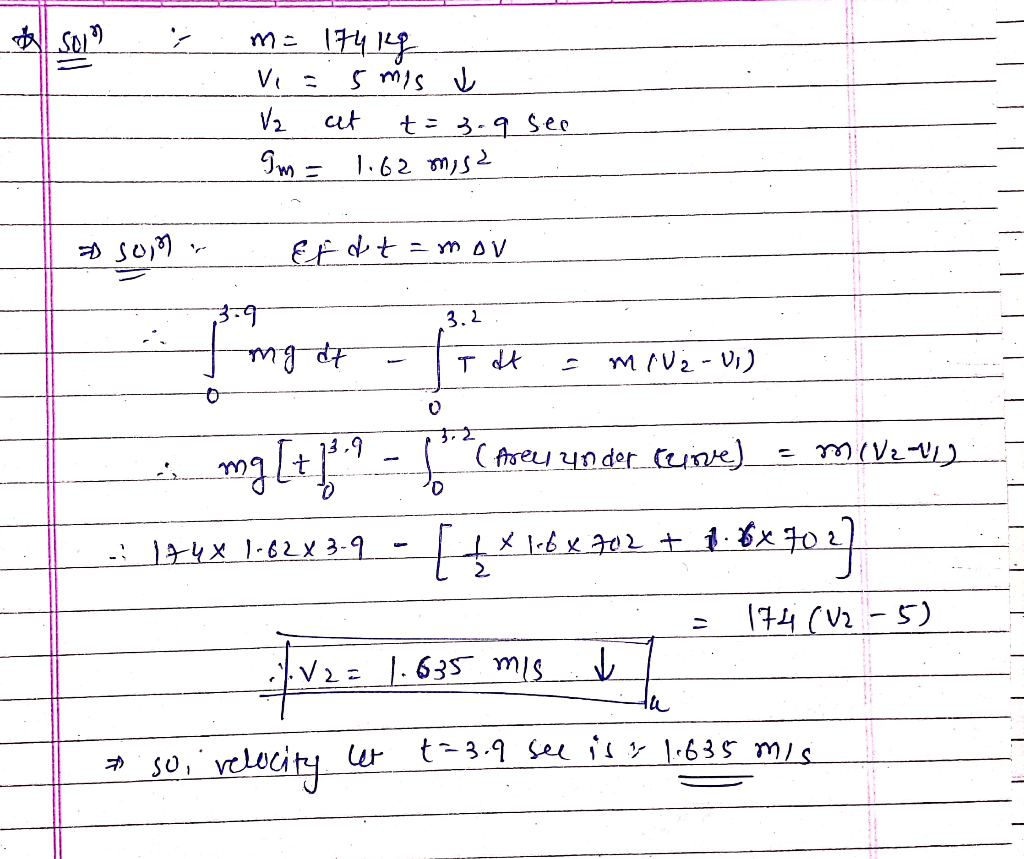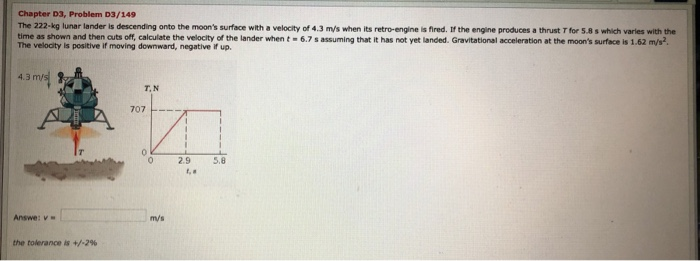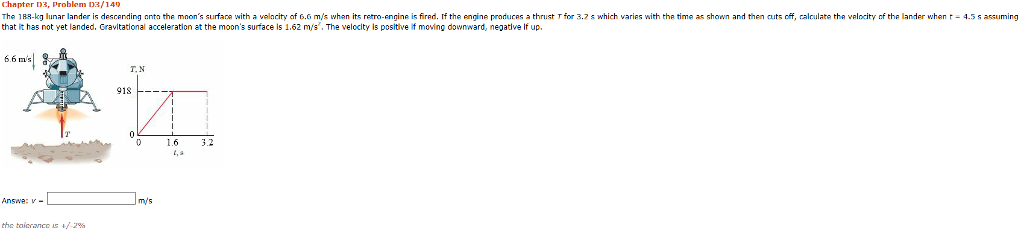Question#### Earn Coins

Coins can be redeemed for fabulous gifts.

Similar Homework Help Questions
• ### Chapter D3, Problem 03/149 The 222-kg lunar lander is descending onto the moon's surface with a...Chapter D3, Problem 03/149 The 222-kg lunar lander is descending onto the moon's surface with a velocity of 4.3 m/s when its retro-engine is fired. If the engine produces a thrust T for 5.8 s which varies with the time as shown and then cuts off, calculate the velocity of the lander when t - 6.7 s assuming that it has not yet landed. Gravitational acceleration at the moon's surface is 1.62 m/s? The velocity is positive if moving downward,...

• ### Chapter D3, Problem D3/140 The 188 g unar lander is descending nnta he mnon's surfan with...Chapter D3, Problem D3/140 The 188 g unar lander is descending nnta he mnon's surfan with a velac y of 6 m 尿when its re o engine ts fired f the engine producas a thrust T or 3.2昴which varies with the that it has not yet landed, Gravitational acceleration at the moon's surface is 1.62 m/s, The velocity is positive if moving downward, negative If up, me as shown and then cuts o calculate the velocity the lander when t...

• ### A lunar lander is descending toward the moon's surface. Until the lander reaches the surface, its...

A lunar lander is descending toward the moon's surface. Until the lander reaches the surface, its height above the surface of the moon is given by y(t)=b−ct+dt^2 , where b = 710m is the initial height of the lander above the surface, c = 64.0m/s, and d = 1.03 m/s^2. (A) What is the initial velocity of the lander, at t = 0? (B) What is the velocity of the lander just before it reaches the lunar surface?

• ### The Apollo Lunar Module was used to make the transition from the spacecraft to the moon's surface and back.

The Apollo Lunar Module was used to make the transition from the spacecraft to the moon's surface and back. Consider a similar module for landing on the surface ofMars. Use conservation of mechanical energy to answer these questions.(a) As the lander is descending, if the pilot decides to shut down the engine when the lander is at a height of 1.8 m, (this may not be a safe height to shut down theengine) and the velocity of the lander (relative...